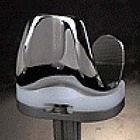# Toward Self-Powered Medical Implants

### Roger Shih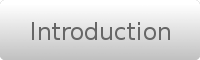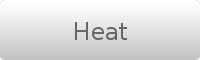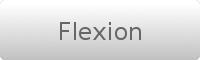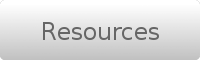[ Introduction ] [ Principle ] [ Device Design ]

## Piezoelectric Ceramics

#### Introduction:

Dr. Stephen R. Platt of the University of Nebraska-Lincoln and Dr. Walied Moussa of the University of Alberta have both designed small self-powered implants for monitoring the structural integrity of artificial joint replacements.  Integrated into the larger joint implant, the monitoring devices are powered by the shifting and compression that occurs in the course of the patient’s daily movements.  The monitors can track an implant’s osseointegration over time, or raise an alert if the joint is on the verge of failure.  While the University of Alberta has not detailed their design’s power-scavenging mechanism, Dr. Platt extensively described the bases for a conceptual design in a 2003 master’s thesis, revealing his power source to be the compression of piezoelectric ceramics.

[top]

#### Principle:

Voltage is produced across piezoelectric materials by forced deformation of the structure, which disrupts the uniform charge distribution therein.  Generation of thousands of volts is often possible by this mechanism.

Piezoelectricity is mathematically described by the coupled equations: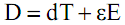Where:
s is strain (m/m)
c is elastic compliance ( (m/m) / (N/m²) ), the reciprocal of elastic modulus
T is stress (N/m²)
d is the piezoelectric strain coefficient ( (m/m) / (V/m) )
E is the electric field (V/m)
D is electric displacement or 2-dimensional charge density (C/m²)
ε is permittivity (F/m)

For electrical open circuit boundary conditions (D = 0), the second equation reduces to: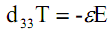Or for a piezoelectric structure,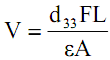Where:
L is thickness
A is cross-sectional area
F is force

At low frequencies piezoelectricity is capacitive, meaning energy E = (1/2)CV².  Therefore, the energy stored by an open-circuit piezoelectric structure under a constant load is given by: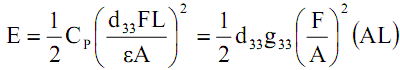[top]

#### Device Design:

Dr. Platt conceived of three piezoelectric stacks which could share load inside the tibial tray of an artificial knee.  A load distribution plate would further help to evenly spread compressive force.  It is emphasized that this design was a conceptual testbed for the suitability of piezoelectrics as a power source under artificial-joint-loading conditions and constraints, rather than a finished product ready for actual implantation.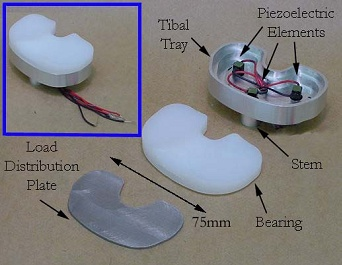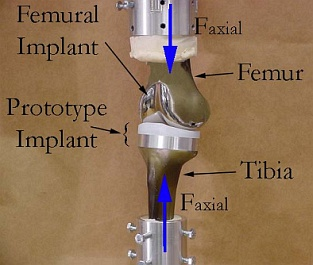Stiff lead zirconate titanate (PZT) ceramics were chosen as the piezoelectric material since the generator structure must be rigid to function as part of the joint.  Two commercially-available options existed: A simple monolithic cylinder measuring 1 cm by 2 cm, and a stack of 145 vertically-polarized wafers 1.8 cm high (available with either 0.5 or 1 cm² base area).  Each slice in the latter structure was wired individually, making them mechanically in series but electrically in parallel.  This structure was eventually chosen since it had better electrical properties for the application.  Whereas the monolithic unit had a high voltage (>10,000 V) and low capacitance (~47 pF), the stack had a more practical voltage (~30 V) and higher capacitance (1-10 μF).  In addition, the stack’s smaller cross-sectional area meant it experienced higher stress for a given force, and the stored energy is proportional to stress squared.  Thus the stack has a greater power output than the monolith despite having a smaller volume.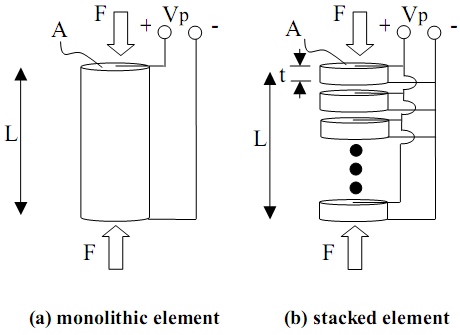One stacked element was stress-tested for 6 million cycles at half the expected operational load, simulating six years of walking.  It was found that electrical output decreased about 4.4% per decade of time.  This sounds fairly impressive, although performance may degrade faster if the load is doubled to better match actual working conditions.

Although the piezoelectric mechanism was capable of generating 4.8 mW of power, theoretically sufficient for the intended applications, one remaining challenge is finding a way to efficiently store the generated energy.  A capacitor is not ideal, since the piezoelectric mechanism itself is capacitive at low frequencies, and energy transfer between capacitors is inefficient.  Dr. Platt believes that the eventual solution will involve high-frequency switching power converters.

[top]Created by Roger Shih for BME 240 at UCI, Spring 2008